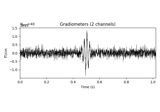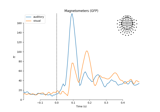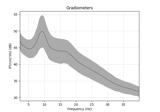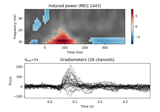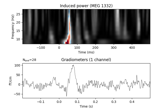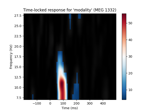# mne.time_frequency.tfr_morlet¶

mne.time_frequency.tfr_morlet(inst, freqs, n_cycles, use_fft=False, return_itc=True, decim=1, n_jobs=1, picks=None, zero_mean=True, average=True, output='power', verbose=None)[source]

Compute Time-Frequency Representation (TFR) using Morlet wavelets.

Same computation as tfr_array_morlet, but operates on Epochs objects instead of NumPy arrays.

Parameters
inst

The epochs or evoked object.

freqsndarray, shape (n_freqs,)

The frequencies in Hz.

n_cyclesfloat | ndarray, shape (n_freqs,)

The number of cycles globally or for each frequency.

use_fftbool, default False

The fft based convolution or not.

return_itcbool, default True

Return inter-trial coherence (ITC) as well as averaged power. Must be False for evoked data.

decimint | slice, default 1

To reduce memory usage, decimation factor after time-frequency decomposition. If int, returns tfr[…, ::decim]. If slice, returns tfr[…, decim].

Note

Decimation may create aliasing artifacts.

n_jobsint

The number of jobs to run in parallel (default 1). Requires the joblib package.

picks

The indices of the channels to decompose. If None, all available good data channels are decomposed.

zero_meanbool, default True

Make sure the wavelet has a mean of zero.

New in version 0.13.0.

averagebool, default True

If False return an EpochsTFR containing separate TFRs for each epoch. If True return an AverageTFR containing the average of all TFRs across epochs.

Note

Using average=True is functionally equivalent to using average=False followed by EpochsTFR.average(), but is more memory efficient.

New in version 0.13.0.

outputstr

Can be “power” (default) or “complex”. If “complex”, then average must be False.

New in version 0.15.0.

verbose

If not None, override default verbose level (see mne.verbose() and Logging documentation for more). If used, it should be passed as a keyword-argument only.

Returns
power

The averaged or single-trial power.

itc

The inter-trial coherence (ITC). Only returned if return_itc is True.

## Examples using mne.time_frequency.tfr_morlet¶